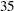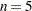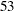//
archives

# SAS Date & Time Functions

This category contains 1 post

### SAS Date, Time, and Datetime Functions

SAS date, time, and datetime functions are used to perform the following tasks:

• compute date, time, and datetime values from calendar and time-of-day values
• compute calendar and time-of-day values from date and datetime values
• convert between date, time, and datetime values
• perform calculations that involve time intervals
• provide information about time intervals

For all interval functions, you can supply the intervals and other character arguments either directly as a quoted string or as a SAS character variable. When you use a character variable, you should set the length of the character variable to at least the length of the longest string for that variable that is used in the DATA step.

Also, to ensure correct results when using interval functions, use date intervals with date values and datetime intervals with datetime values.

See SAS Language Reference: Dictionary for a complete description of these functions.

The following list shows SAS date, time, and datetime functions in alphabetical order.

DATE()

returns today’s date as a SAS date value.

DATEJUL( yyddd )

returns the SAS date value when given the Julian date in yyddd or yyyyddd format. For example, DATE = DATEJUL(99001); assigns the SAS date value ’01JAN99’D to DATE, and DATE = DATEJUL(1999365); assigns the SAS date value ’31DEC1999’D to DATE.

DATEPART( datetime )

returns the date part of a SAS datetime value as a date value.

DATETIME()

returns the current date and time of day as a SAS datetime value.

DAY( date )

returns the day of the month from a SAS date value.

DHMS( date, hour, minute, second )

returns a SAS datetime value for date, hour, minute, and second values.

HMS( hour, minute, second )

returns a SAS time value for hour, minute, and second values.

HOLIDAY( ‘holiday‘, year )

returns a SAS date value for the holiday and year specified. Valid values for holiday are ‘BOXING’, ‘CANADA’, ‘CANADAOBSERVED’, ‘CHRISTMAS’, ‘COLUMBUS’, ‘EASTER’, ‘FATHERS’, ‘HALLOWEEN’, ‘LABOR’, ‘MLK’, ‘MEMORIAL’, ‘MOTHERS’, ‘NEWYEAR’,’THANKSGIVING’, ‘THANKSGIVINGCANADA’, ‘USINDEPENDENCE’, ‘USPRESIDENTS’, ‘VALENTINES’, ‘VETERANS’, ‘VETERANSUSG’, ‘VETERANSUSPS’, and ‘VICTORIA’. For example: EASTER2000 = HOLIDAY(’EASTER’, 2000);

HOUR( datetime )

returns the hour from a SAS datetime or time value.

INTCINDEX( ‘date-interval‘, date )

INTCINDEX( ‘datetime-interval‘, datetime )

returns the index of the seasonal cycle when given an interval and an appropriate SAS date, datetime, or time value. For example, the seasonal cycle for INTERVAL=’DAY’ is ‘WEEK’, soINTCINDEX(’DAY’,’01SEP78’D); returns 35 because September 1, 1978, is the sixth day of theth week of the year. For correct results, date intervals should be used with date values, and datetime intervals should be used with datetime values.

INTCK( ‘date-interval‘, date1, date2 <, ‘method’> )

INTCK( ‘datetime-interval‘, datetime1, datetime2 <, ‘method’> )

returns the number of boundaries of intervals of the given kind that lie between the two date or datetime values. The optional method argument specifies that the intervals are counted using either a discrete or a continuous method. The default DISCRETE (or DISC or D) method uses discrete time intervals. For the DISCRETE method, the distance in MONTHS between January 31, 2000, and February 1, 2000, is one month. The CONTINUOUS (or CONT or C) method uses continuous time intervals. For the CONTINUOUS method, the distance in MONTHS between January 15, 2000, and February 14, 2000, is zero, but the distance in MONTHS between January 15, 2000, and February 15, 2000, is one month.

INTCYCLE( ‘interval‘ <, seasonality> )

returns the interval of the seasonal cycle, given a date, time, or datetime interval. For example, INTCYCLE(‘MONTH’) returns ‘YEAR’ because the months January, February, …, December constitute a yearly cycle. INTCYCLE(‘DAY’) returns ‘WEEK’ because Sunday, Monday, …, Saturday constitute a weekly cycle.

You can specify the optional seasonality argument to construct a cycle other than the default seasonal cycle. For example, INTCYCLE(‘MONTH’, 3) returns ‘QTR’. The optional second argument is the seasonal frequency.

INTFIT( date1, date2, ‘D‘ )

INTFIT( datetime1, datetime2, ‘DT‘ )

INTFIT( obs1, obs2, ‘OBS‘ )

returns an interval that fits exactly between two SAS date, datetime, or observation values. That is, if the interval result of the INTFIT function is used with date1,, and SAMEDAY alignment in the INTNX function, then the result is date2. This concept is illustrated in the following example, where result1 is the same as date1 and result2 is the same as date2.

```   FitInterval = INTFIT( date1, date2, 'D' );
result1 = INTNX( FitInterval, date1, 0, 'SAMEDAY');
result2 = INTNX( FitInterval, date1, 1, 'SAMEDAY');
```

More than one interval can fit the preceding definition. For example, two SAS date values that are seven days apart could be fit with either ‘DAY7’ or ‘WEEK’. The INTFIT function chooses the more common interval, so ‘WEEK’ is the result when the dates are seven days apart. The INTFIT function can be used to detect the possible frequency of the time series or to analyze frequencies of other events in a time series, such as outliers or missing values.

INTFMT(‘interval‘ ,’size‘)

returns a recommended format when given a date, time, or datetime interval for displaying the time ID values associated with a time series of the given interval. The second argument to INTFMT controls the width of the year for date formats; it can take the value ‘long’ or ‘l’ to specify that the returned format display a four-digit year or the value ‘short’ or ‘s’ to specify that the returned format display a two-digit year.

INTGET( date1, date2, date3 )

INTGET( datetime1, datetime2, datetime3 )

returns an interval that fits three consecutive SAS date or datetime values. The INTGET function examines two intervals: the first interval between date1 and date2, and the second interval between date2 and date3. In order for an interval to be detected, either the two intervals must be the same or one interval must be an integer multiple of the other interval. That is, INTGET assumes that at least two of the dates are consecutive points in the time series, and that the other two dates are also consecutive or represent the points before and after missing observations. The INTGET function assumes that large values are SAS datetime values, which are measured in seconds, and that smaller values are SAS date values, which are measured in days. The INTGET function can be used to detect the possible frequency of the time series or to analyze frequencies of other events in a time series, such as outliers or missing values.

INTINDEX( ‘date-interval‘, date <, seasonality> )

INTINDEX( ‘datetime-interval‘, datetime <, seasonality> )

returns the seasonal index for the specified date or datetime interval and an appropriate date or datetime value. The seasonal index is a number that represents the position of the date or datetime value in the seasonal cycle of the specified interval. For example, INTINDEX(’MONTH’,’01DEC2000’D); returns 12 because monthly data is yearly periodic and DECEMBER is theth month of the year. However, INTINDEX(’DAY’,’01DEC2000’D); returns 6 because daily data is weekly periodic and December 01, 2000, is a Friday, the sixth day of the week. To correctly identify the seasonal index, the interval specification should agree with the date or datetime value. For example, INTINDEX(’DTMONTH’,’01DEC2000’D); and INTINDEX(’MONTH’,’01DEC2000:00:00:00’DT);do not return the expected value of 12. However, both INTINDEX(’MONTH’,’01DEC2000’D); and INTINDEX(’DTMONTH’,’01DEC2000:00:00:00’DT); return the expected value of 12.

You can specify the optional seasonality argument to use a seasonal cycle other than the default seasonal cycle. For example, INTINDEX(’MONTH’,’01APR2000’D); returns the value 4, to indicate the fourth month of the year. However, INTINDEX(’MONTH’,’01APR2000’D,3); and INTINDEX(’MONTH’,’01APR2000’D,’QTR’); return the value 1 to indicate the first month of the quarter. Specifying either 3 or ‘QTR’ for the third argument uses a quarterly seasonal cycle instead of the default yearly seasonal cycle.

INTNX( ‘date-interval‘, date, n <, ‘alignment’> )

INTNX( ‘datetime-interval‘, datetime, n <, ‘alignment’> )

returns the date or datetime value of the beginning of the interval that is n intervals from the interval that contains the given date or datetime value. The optional alignment argument specifies that the returned date is aligned to the beginning, middle, or end of the interval. Beginning is the default. In addition, you can specify SAME (S) alignment. The SAME alignment bases the alignment of the calculated date or datetime value on the alignment of the input date or datetime value. As illustrated in the following example, the SAME alignment can be used to calculate the meaning of “same day next year” or “same day two weeks from now.”

```   nextYear = INTNX( 'YEAR', '15Apr2007'D, 1, 'S' );
TwoWeeks = INTNX( 'WEEK', '15Apr2007'D, 2, 'S' );
```

The preceding example returns ’15Apr2008’D for nextYear and ’29Apr2007’D for TwoWeeks.

For all values of alignment, the number of discrete intervals n between the input date and the resulting date agrees with the input value. In the following example, the result is always that n2 = n1:

```   date2 = INTNX( interval, date1, n1, align );
n2 = INTCK( interval, date1, date2 );
```

The preceding example uses the DISCRETE method of the INTCK function by default. The result n2 = n1 does not always apply when the CONTINUOUS method of the INTCK function is specified.

INTSEAS( ‘interval‘ <, seasonality> )

returns the length of the seasonal cycle for the specified date or datetime interval. The length of a seasonal cycle is the number of intervals in a seasonal cycle. For example, when the interval for a time series is described as monthly, many procedures use the option INTERVAL=MONTH to indicate that each observation in the data corresponds to a particular month. Monthly data are considered to be periodic for a one-year seasonal cycle. There are 12 months in one year, so the number of intervals (months) in a seasonal cycle (year) is 12. For quarterly data, there are 4 quarters in one year, so the number of intervals in a seasonal cycle is 4. The periodicity is not always one year. For example, INTERVAL=DAY is considered to have a seasonal cycle of one week, and because there are 7 days in a week, the number of intervals in a seasonal cycle is 7.

You can specify the optional seasonality argument to use a seasonal cycle other than the default seasonal cycle. For example, INTSEAS(‘MONTH’, 3) and INTSEAS(‘MONTH’, ‘QTR’) both specify a quarterly seasonal cycle and return the value 3. If the optional seasonality argument is numeric, it is the seasonal frequency. If the optional seasonality argument is character, it is the seasonal cycle.

INTSHIFT( ‘interval‘ )

returns the shift interval that applies to the shift index if a subperiod is specified. For example, YEAR intervals are shifted by MONTH, so INTSHIFT(‘YEAR’) returns ‘MONTH’.

INTTEST( ‘interval‘ )

returns 1 if the interval name is valid, 0 otherwise. For example, VALID = INTTEST(’MONTH’); should set VALID to 1, while VALID = INTTEST(’NOTANINTERVAL’); should set VALID to 0. The INTTEST function can be useful in verifying which values of multiplier n and the shift index s are valid in constructing an interval name.

JULDATE( date )

returns the Julian date from a SAS date value. The format of the Julian date is either yyddd or yyyyddd depending on the value of the system option YEARCUTOFF=. For example, using the default system option values, JULDATE( ’31DEC1999’D ); returns 99365, while JULDATE( ’31DEC1899’D ); returns 1899365.

MDY( month, day, year )

returns a SAS date value for month, day, and year values.

MINUTE( datetime )

returns the minute from a SAS time or datetime value.

MONTH( date )

returns the numerical value for the month of the year from a SAS date value. For example, MONTH=MONTH(’01JAN2000’D); returns, the numerical value for January.

NWKDOM( n, weekday, month, year )

returns a SAS date value for theth weekday of the month and year specified. For example, Thanksgiving is always the fourth (n=4) Thursday (weekday=5) in November (month=11). ThusTHANKS2000 = NWKDOM( 4, 5, 11, 2000); returns the SAS date value for Thanksgiving in the year 2000. The last weekday of a month can be specified by using n=5. Memorial Day in the United States is the last (n=5) Monday (weekday=2) in May (month=5), and so MEMORIAL2002 = NWKDOM( 5, 2, 5, 2002); returns the SAS date value for Memorial Day in 2002. Becausealways specifies the last occurrence of the month and most months have only 4 instances of each day, the result foris often the same as the result for. NWKDOM is useful for calculating the SAS date values of holidays that are defined in this manner.

QTR( date )

returns the quarter of the year from a SAS date value.

SECOND( date )

returns the second from a SAS time or datetime value.

TIME()

returns the current time of day.

TIMEPART( datetime )

returns the time part of a SAS datetime value.

TODAY()

returns the current date as a SAS date value. (TODAY is another name for the DATE function.)

WEEK( date <, ‘descriptor’> )

returns the week of year from a SAS date value. The algorithm used to calculate the week depends on the descriptor, which can take the value ‘U’, ‘V’, or ‘W’.

If the descriptor is ‘U,’ weeks start on Sunday and the range isto. If weeksandexist, they are only partial weeks. Week 52 can be a partial week.

If the descriptor is ‘V’, the result is equivalent to the ISO 8601 week of year definition. The range isto. Weekis a leap week. The first week of the year, Week, and the last week of the year, Weekor, can include days in another Gregorian calendar year.

If the descriptor is ‘W’, weeks start on Monday and the range isto. If weeksandexist, they are only partial weeks. Week 52 can be a partial week.

WEEKDAY( date )

returns the day of the week from a SAS date value. For example WEEKDAY=WEEKDAY(’17OCT1991’D); returns, the numerical value for Thursday.

YEAR( date )

returns the year from a SAS date value.

YYQ( year, quarter )

returns a SAS date value for year and quarter values.